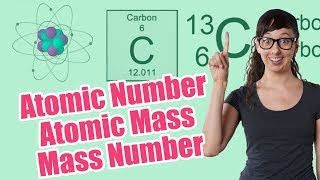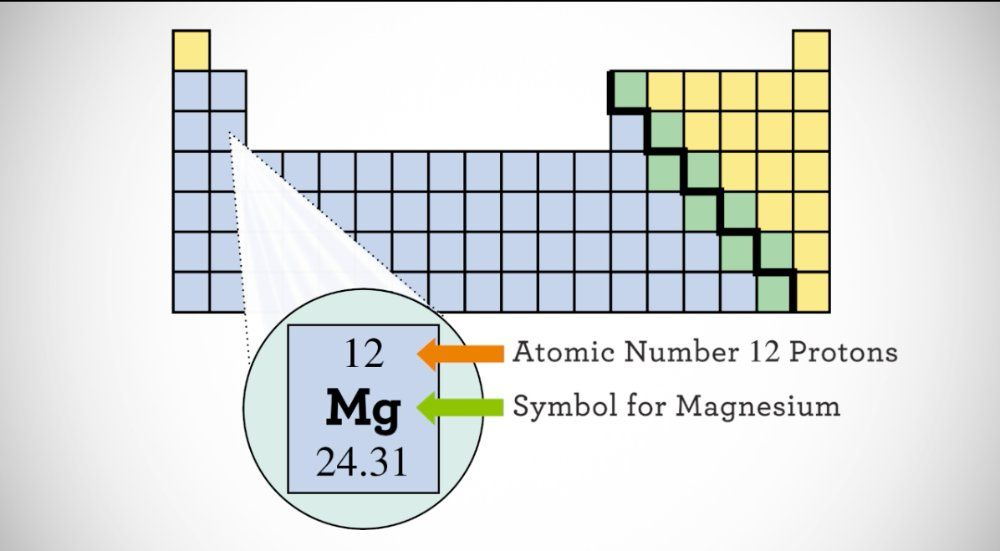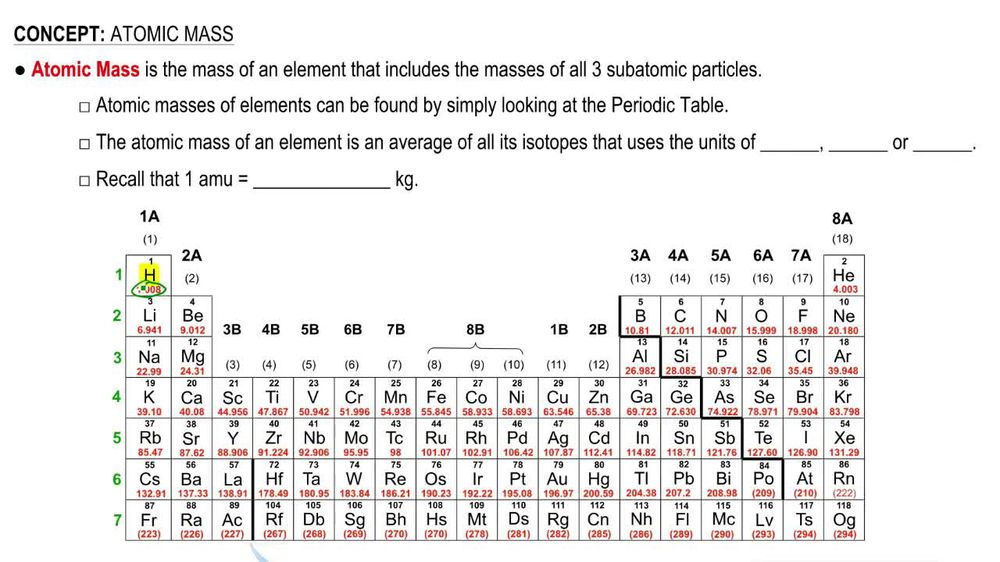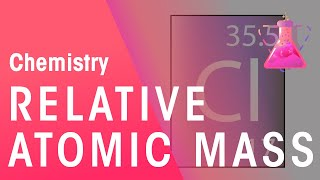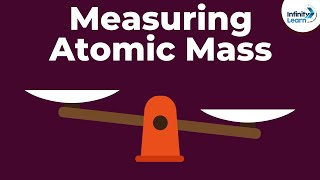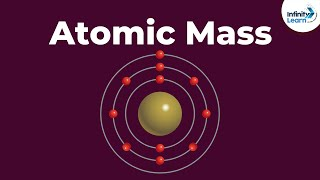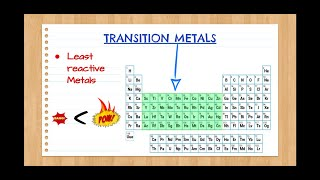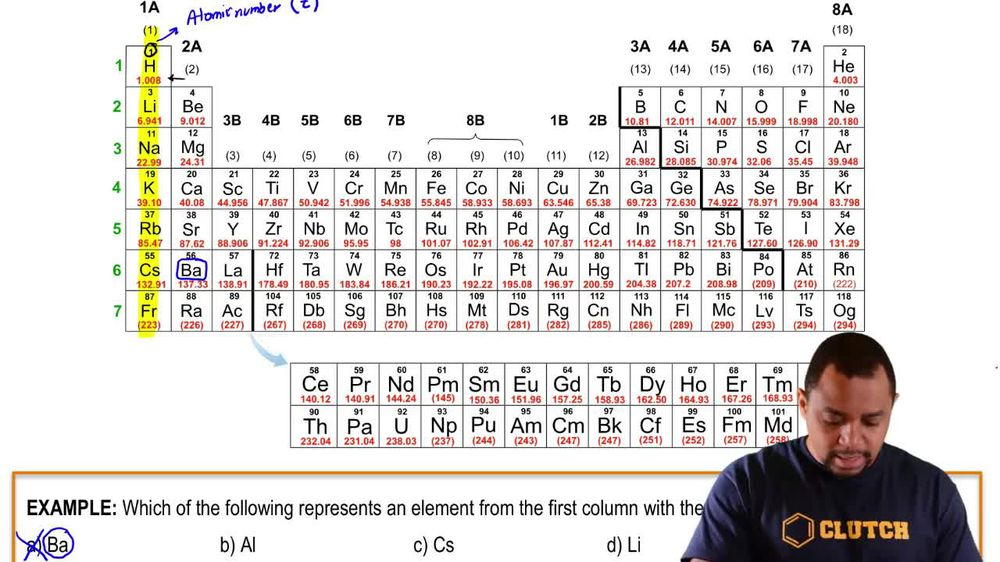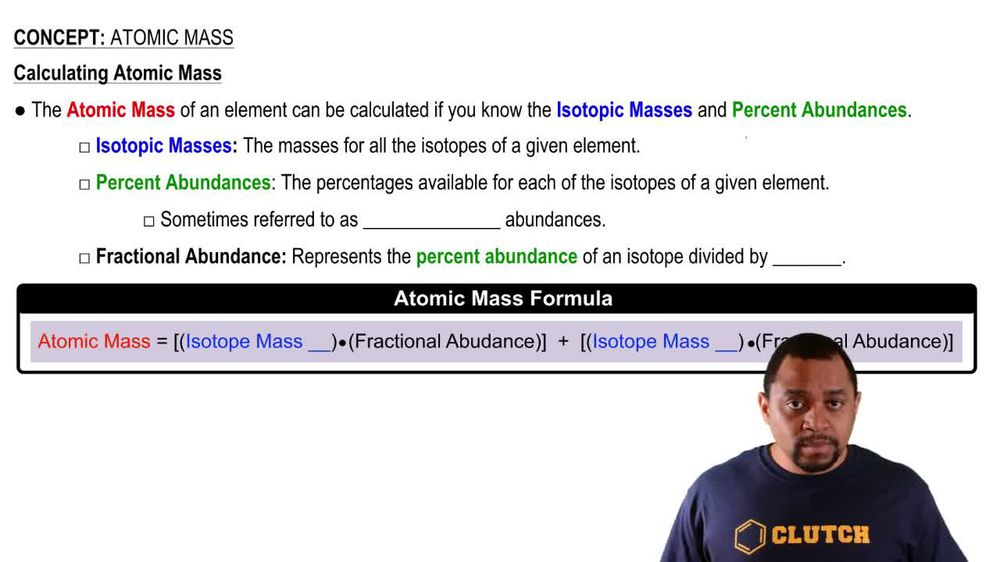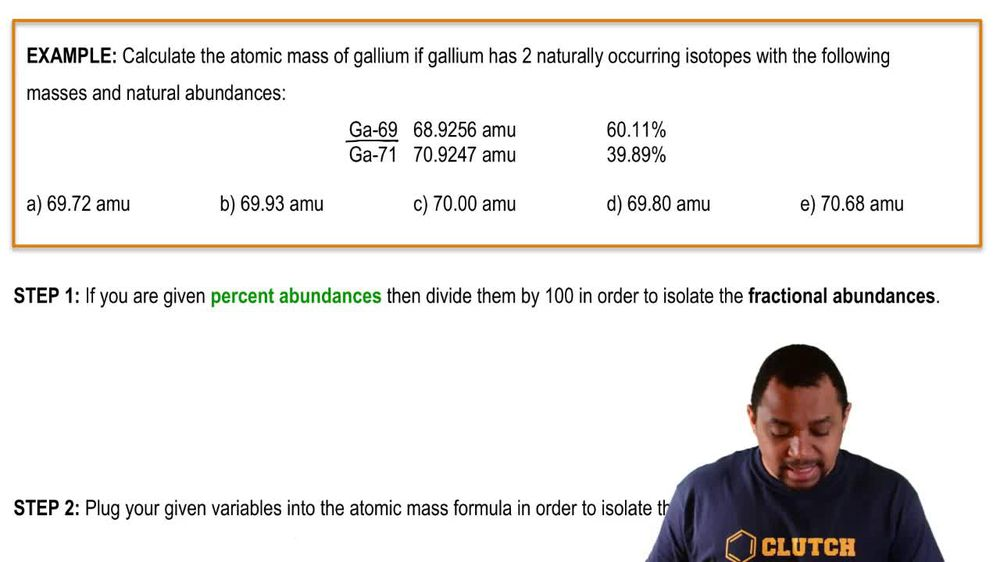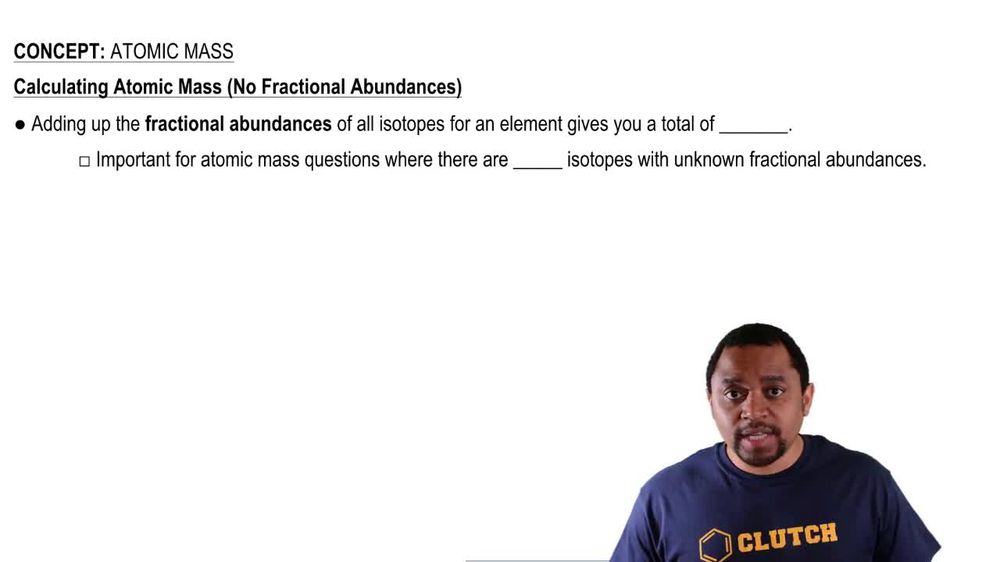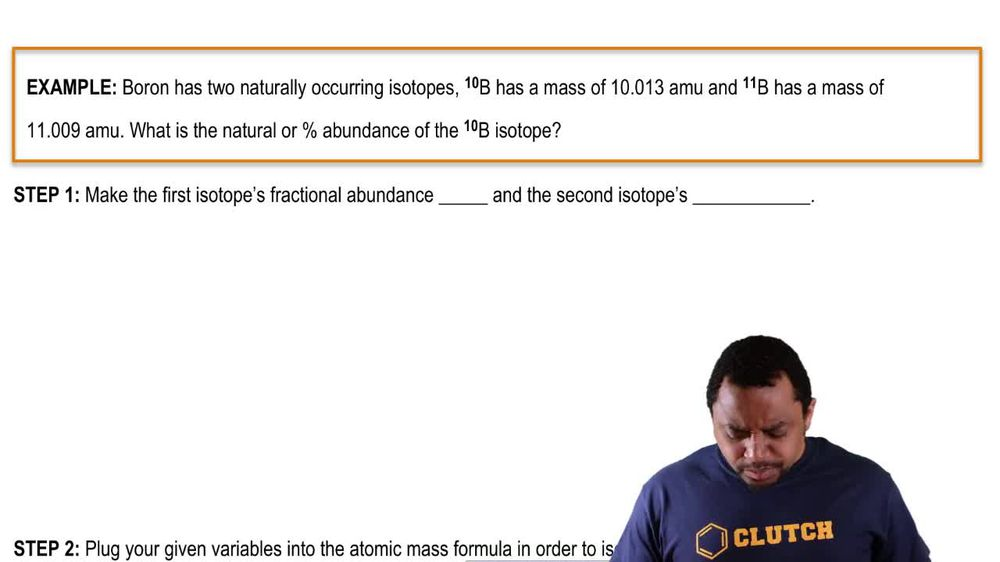Start typing, then use the up and down arrows to select an option from the list.
1. 2. Atoms & Elements2. Atomic Mass
Problem

# Bromine has two naturally occurring isotopes, bromine-79 1atomic mass = 78.9183 u; abundance = 50.69%2 a n d bromine-81 1atomic mass = 80.9163 u; abundance = 49.31%2. Calculate the atomic weight of bromine.

Relevant Solution3m
Play a video:
Hey everyone in this example, we're given two isotopes of potassium and we need to find the average atomic way of a potassium atom. If the heavier isotope has an abundance of 6.7302%. We should recall that to find average atomic mass of an atom. We're going to take the some of the atomic weights of of we should say and elements isotopes according to their percent abundance. So to find our calculation, we're going to get that our atomic weight or rather average atomic weight of our adam potassium Is going to be equal to first. Our atomic weight of our potassium 39 isotope is given in the prompt as 38.963706 Am use we are not given its percent abundance were only given the percent abundance of potassium as 6.7302%. So because we don't know the percent abundance of potassium 39 We're going to multiply it by the difference between one or 100% of our isotope minus the percent abundance of potassium 41. The other isotope As a decimal. So we should convert this to a decimal by dividing by 100 and we would have .067302. And sorry, that should be in view. So we would have .067302 converting the% 41 to a decimal. So we would plug that in here and then we're going to again add the sum of all the atomic weights of our isotopes of potassium. So we're going to add this two and I'm just going to make more room. Our atomic weight of potassium 41 given in the prompt as .961825 AM use and we're going to multiply this by the abundance given in the prompt for this isotope Which is given in the prompt as again . as a decimal here. So typing this into our calculators what we should have first taking one minus our decimal here and then multiplying this value of our parentheses by our atomic weight of potassium 39 we're going to get a value of 36.3414 am use And we're going to add this to the product of our atomic weight of potassium to its percent abundance as a decimal. Which is going to give us a value of 2.75681 am use And adding these two values up. We're going to get a final result of 39.0982 am use as our average atomic weight of our atom potassium And this will be our final answer to complete this example. So I hope that everything I explained was clear. If you have any questions please leave them down below and I will see everyone in the next practice video